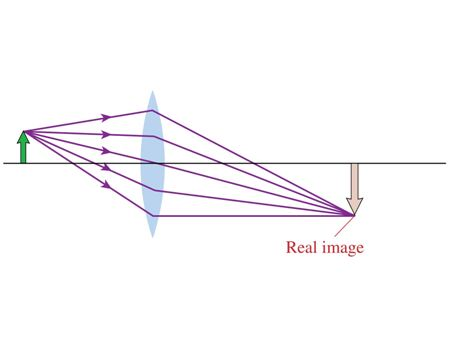# Problem: A lens creates an image as shown. In this situation, the object distance s isa) Larger than the focal length f. ?b) Smaller than focal length f. ?c) Equal to the focal length f. ?

###### FREE Expert Solution

The lens maker equation:

$\overline{)\frac{\mathbf{1}}{{\mathbf{s}}_{\mathbf{o}}}{\mathbf{+}}\frac{\mathbf{1}}{{\mathbf{s}}_{\mathbf{i}}}{\mathbf{=}}\frac{\mathbf{1}}{\mathbf{f}}}$

Considering distances on the lens to be negative:

so = - so and si = si

1/f =1/si - 1/so

88% (49 ratings)###### Problem Details

A lens creates an image as shown. In this situation, the object distance s isa) Larger than the focal length f. ?

b) Smaller than focal length f. ?

c) Equal to the focal length f. ?